# ISTEXT Function

Tests if a given value is a text string or not

## What is the ISTEXT Function?

The ISTEXT Function is categorized under Excel Information functions. The function will test if a given value is a text string or not. If the given value is text, it will return TRUE, or if not, it will return FALSE.

In doing financial analysis, if we want a particular file to input only text values in a designated cell, using this function along with data validation will help us do that.

### Formula

=ISTEXT(value)

The ISTEXT function uses only one argument:

1. Value (required argument) – This is the given value or expression that we wish to test. The value argument can be a blank (i.e., an empty cell), an error, a logical value, a text, a  number, a reference value, or a name referring to any of these.

### How to use the ISTEXT Function in Excel?

As a worksheet function, ISTEXT can be entered as part of a formula in a cell of a worksheet.

To understand the uses of the ISTEXT function, let’s consider a few examples:

#### Example 1

Suppose we are given the following data: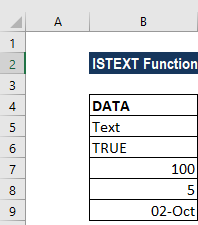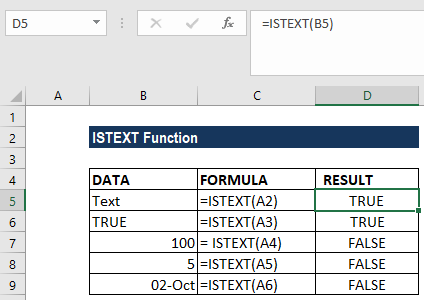As we see above, when we apply the ISTEXT formula, we get the result in the form of TRUE or FALSE.

#### Example 2

Suppose we wish to allow only text to be entered in a particular cell. In such a scenario, we can use data validation along with ISTEXT to get the desired results.

Using the data below: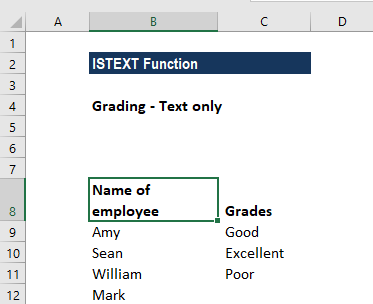We want only text to be entered in column C9:C12, so we apply data validation to the cells. The formula to be used would be =ISTEXT(B9).

How to apply this formula?

We need to go to the Data tab. Under the Data tab, click on Data Validation, then click on Validation criteria and select “Custom”. Use a formula to determine which cells to format. For this example, we selected column B6:B10: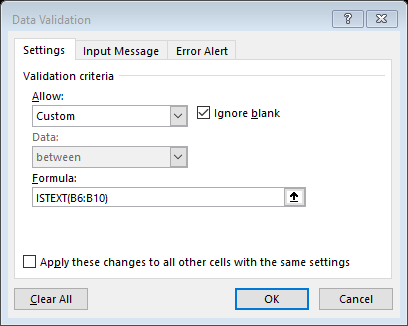If we want, we can input an error message. Click on Input Message and type the message on the Input message box.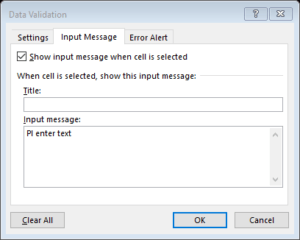Now, click OK.

Data validation rules are now triggered when any user adds or changes a cell value. Cell references in data validation formulas are checked for rules defined in data validation.

The ISTEXT function returns TRUE when a value is text and FALSE if it is not. As a result, all text input will pass validation, but numbers and formulas will fail validation.

We get the results below when we try to enter numbers: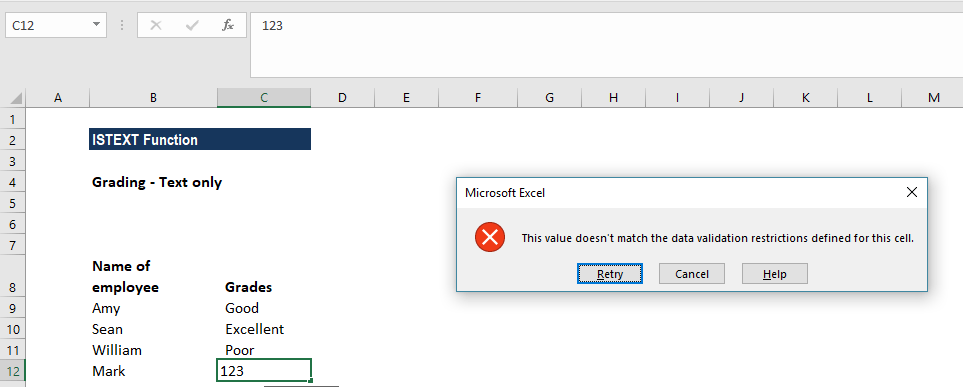#### Example 3

We can also ensure valid text is entered by using ISTEXT with the IF function. This can be done using a nesting formula. Suppose we have a form that is to be filled out by users. The form is being sent to various users. We want to ensure that only names are entered in cell B3. For this, we will use the formula =IF(B3=””,”Enter Your Name”,IF(ISTEXT(B3),”Valid Name”,”Please Enter A Valid Name”)), as shown below: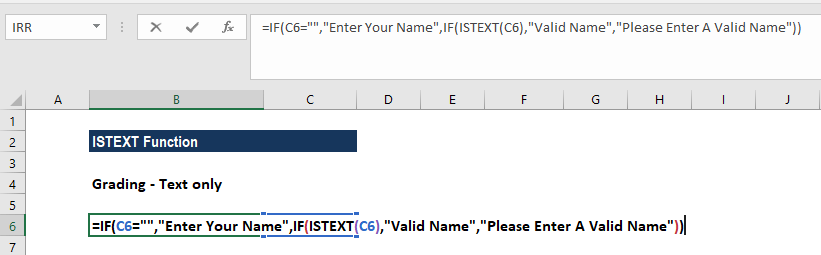If we try to input a number, we will get an error, while a valid name will be accepted, as shown below.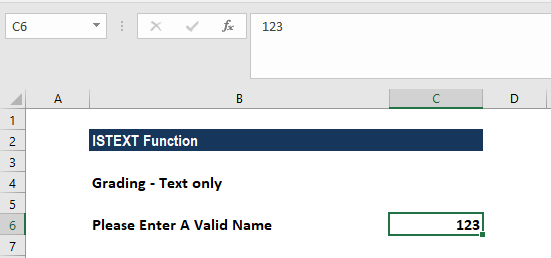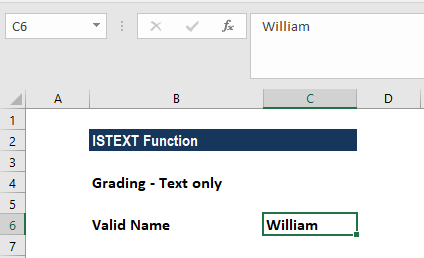### A few things to remember about the ISTEXT Function

1. The ISTEXT function is available in MS Excel 2000 and later versions.
2. The function will return FALSE even for formula errors such as #VALUE!, #NULL!, etc.
3. ISTEXT belongs to the IS family. We can use an IS function to get information about a value before performing a calculation or other action on it.

Thanks for reading CFI’s guide to important Excel functions! By taking the time to learn and master these functions, you’ll significantly speed up your financial analysis. To learn more, check out these additional CFI resources:

• Excel Finance Functions